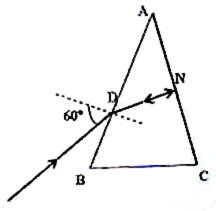## Thursday, October 30, 2008

### Geometrical Optics- Questions (MCQ) on Refraction at Prisms

Questions involving the refraction produced by prisms often find place in Medical, Engineering and other Degree Entrance Exam question papers. Here are two questions in this section:

(1) The face AC of a glass prism of angle 30º is silvered. A ray of light incident at an angle of 60º on face AB retraces its path on getting reflected from the silvered face AC. If the face AC is not silvered, the deviation that can be produced by the prism will be(a) 0º

(b) 30º

(c) 45º

(d) 60º

(e) 90º

The deviation (d) produced by a prism is given by

d = i1 + i2 A where i1 and i2 are respectively the angle of incidence and the angle of emergence and A is the angle of the prism. Here we have i1 = 60º, i2 = 0º (since the ray falling normally will proceed undeviated from the face AC if it is not silvered) and A = 60º.

Therefore, d = 30º.

(2) In the above question, what is the refractive index of the material of the prism?

(a) 1.732

(b) 1.652

(c) 1.667

(d) 1.5

(e) 1.414

In the triangle ADN angle AND is 60º since angle DAN = 30º and angle DNA = 90º. Therefore, the angle of refraction at D is 30º. The refractive index of the material of the lens (n) is given by

n = sin i1/ sinr1 = sin 60º/ sin 30º = √3 = 1.732

(3) Two thin (small angled) prisms are combined to produce dispersion without deviation. One prism has angle 5º and refractive index 1.56. If the other prism has refractive index 1.7, what is its angle?

(a) 3º

(b) 4º

(c) 5º

(d) 6º

(e)

Since the deviation (d) produced by a small angled prism of angle A and refractive index n is given by

d = (n – 1)A, the condition for dispersion without deviation on combining two prisms of angles A1 and A2 with refractive indices n1 and n2 respectively is

(n1 – 1)A1 = (n2 – 1)A2

Therefore, 0.56×5 = 0.7×A2 so that A2 = 4º

On this site you will find many questions (with solution) on refraction at plane surfaces as well as at curved surfaces. To access all of them type in ‘refraction’ in the search box at the top left side of this page and click on the adjacent ‘search blog’ box.